# 一、前言

ICLR作为机器学习方向的顶会，最近看了ICLR2023 Openreview的论文投稿分析，通过2022和2023年论文关键词、标题高频词等信息的可视化比较。根据前十的关键词频率排名频率来看，基本上和去年保持一致，大火的领域依旧大火。但是可以明显看到前五名关键词的频率差距逐渐减少。 有意思的是representation learning这一关键词终于又重回前三，再次为「国际学习表征会议」（ICLR）正名。graph neural network这一关键词则是掉了一名，与representation learning交换了位置，但相比于去年的频率仍然火爆。GCN作为GNN的变种，依然是一个发论文的热门。

Keyword 2022 2023
reinforcement learning 1 1
deep learning 2 2
representation learning 4 3
graph neural network 3 4
transformer 5 5
federate learning 7 6
self-supervised learning 6 7
contrastive learning 10 8
robustness 9 9
generative model 8 10

Title 2022 2023
representation 1 1
graph 3 2
data 6 3
reinforcement 2 4
transformer 7 5
training 5 6
image 10 7
efficient 9 8
language 15 9
federate 14 10

# 二、图的概念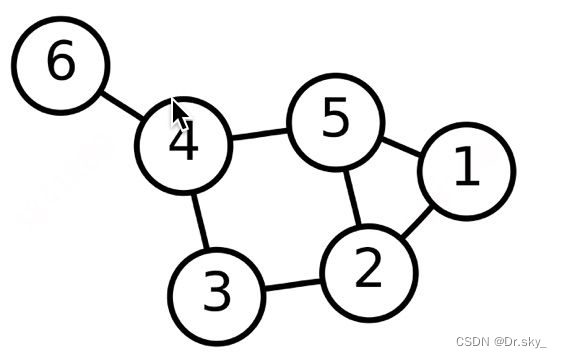# 三、GNN图神经网络

GNN全称—-图神经网络，它是一种直接作用于图结构上的神经网络。我们可以把图中的每一个节点 V 当作个体对象，而每一条边 E 当作个体与个体间的某种联系，所有节点组成的关系网就是最后的图 U。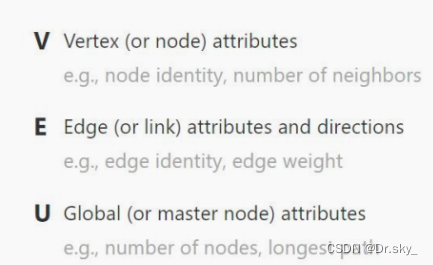E的元素都是二元组，用(x,y)表示，其中x,y∈V。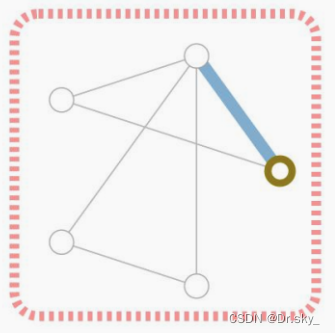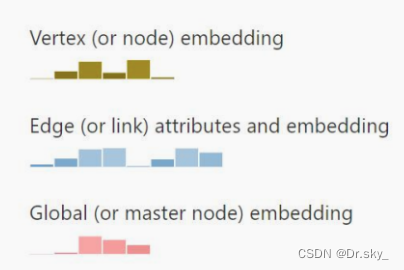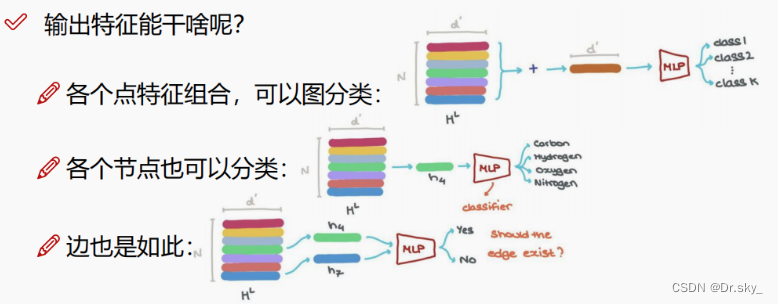GNN的主要目的就是用图结构提取特征，最终是做节点分类还是关联预测由我们自己决定。

# 五、GNN原理

## 5.1 邻接矩阵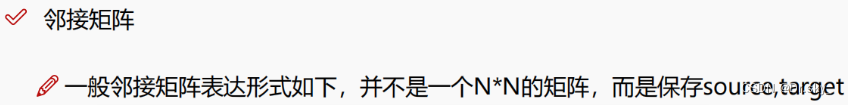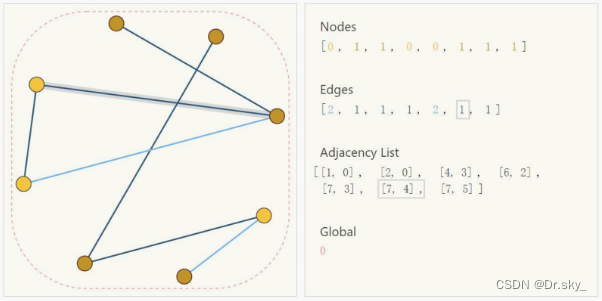## 5.2 聚合操作

GNN的输入一般是每个节点的起始特征向量和表示节点间关系的邻接矩阵，有了这两个输入信息，接下来就是聚合操作了。所谓的聚合，其实就是将周边与节点 Vi 有关联的节点{Va , Vb , . . .}加权到Vi上，当作一次特征更新。同理，对图中的每个节点进行聚合操作，更新所有图节点的特征。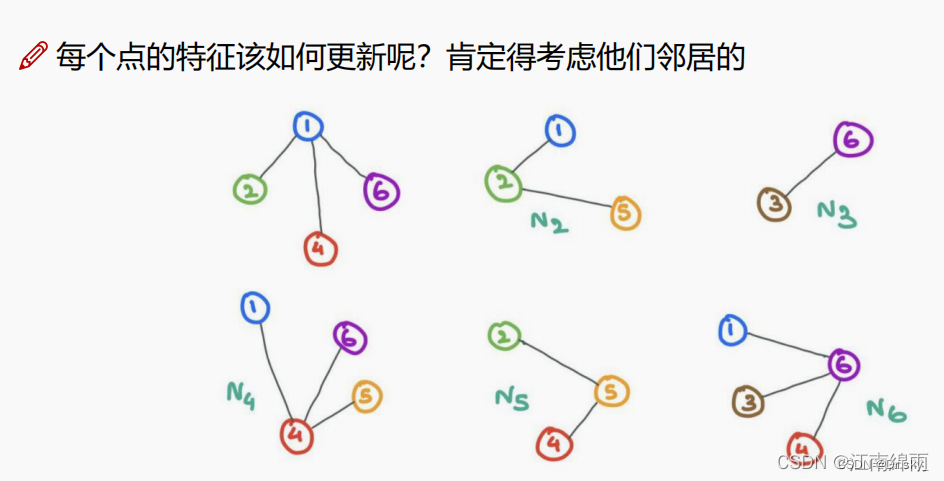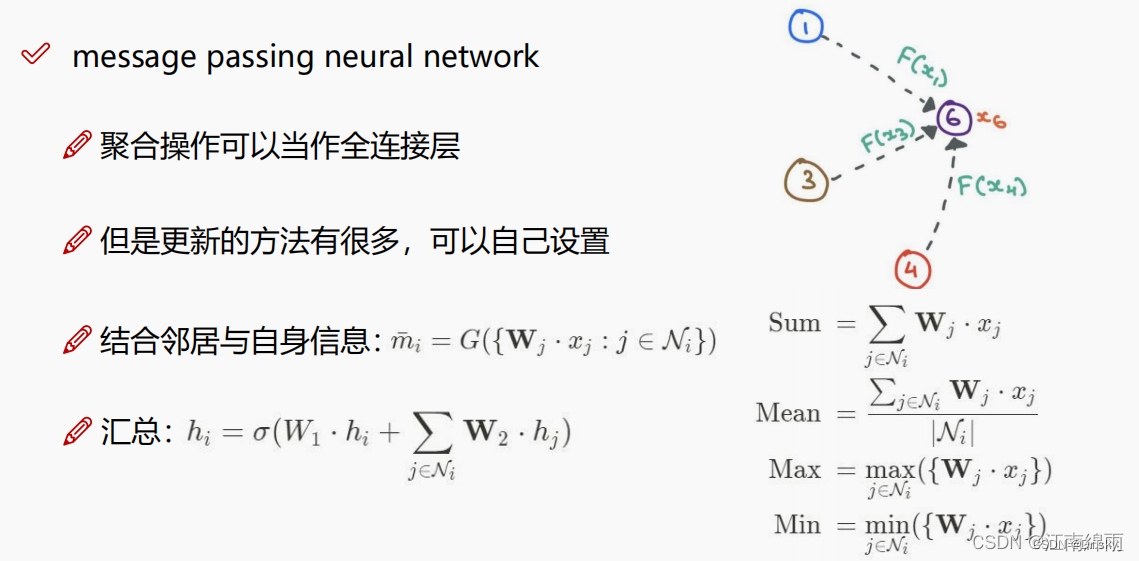## 5.3 多层迭代

CNN，RNN都可以有多个层，那么GNN也当然可以。一次图节点聚合操作与 w ww 加权，可以理解为一层，后面再重复进行聚合、加权，就是多层迭代了。一般GNN只要3~5层即可，所以训练GNN对算力要求很低。如下图所示：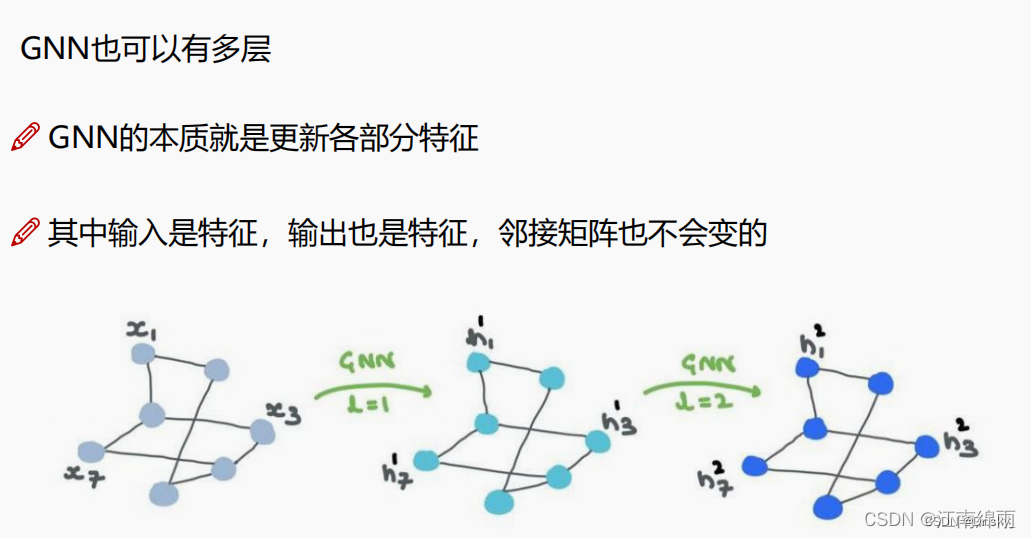# 六、GCN图卷集神经网路

（）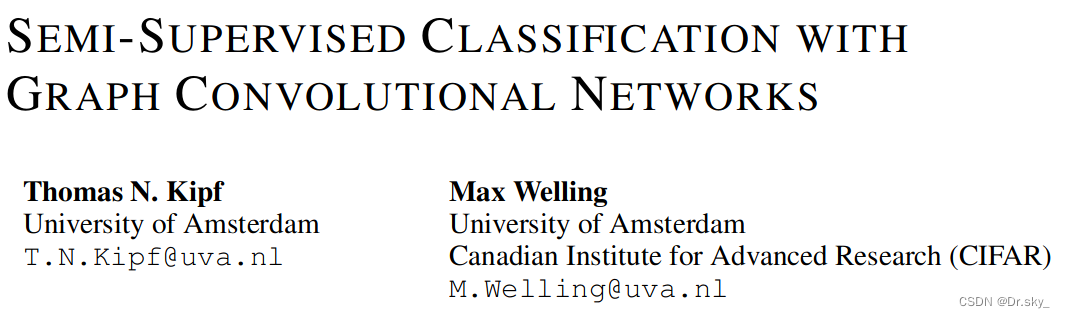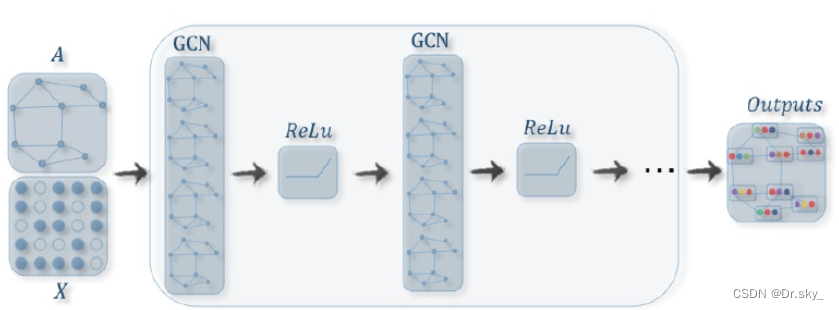GCN的核心部分是什么样子：

Labeled graph（标签图），图的路径和节点标签

Degree matrix（度矩阵） ，每个节点的度，即与每个节点连接的节点数量

Laplacian matrix（拉普拉斯矩阵），类比离散拉普拉斯算子定义图上拉普拉斯算子为节点与邻居节点特征信息f差异的和

L = D – A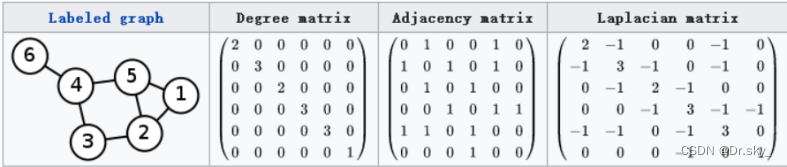GCN也是一个层，它的层与层之间的传播方式是：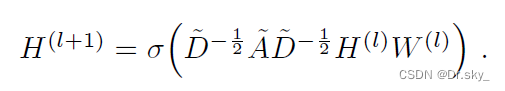• A波浪=A+I，I是单位矩阵
• D波浪是A波浪的度矩阵（degree matrix），公式为
• H是每一层的特征，对于输入层的话，H就是X
• σ是非线性激活函数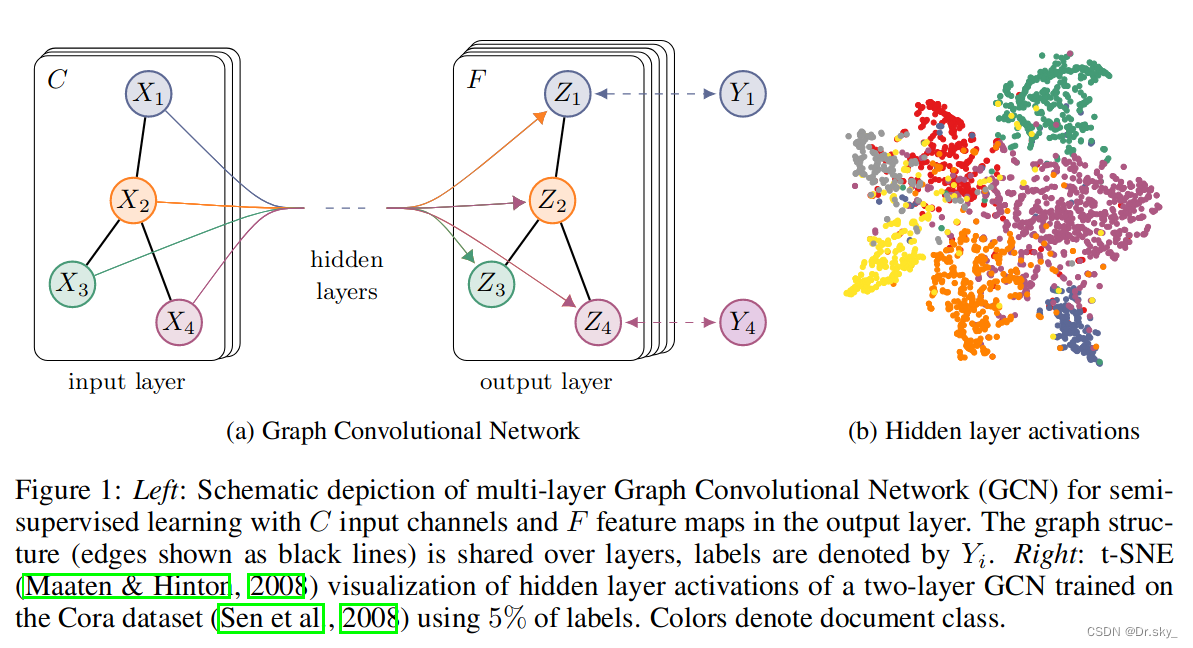上图中的GCN输入一个图，通过若干层GCN每个node的特征从X变成了Z，但是，无论中间有多少层，node之间的连接关系，即A，都是共享的。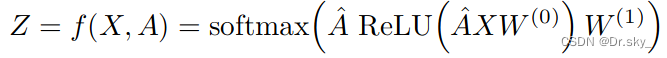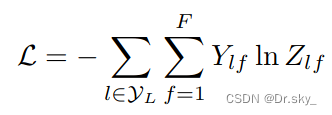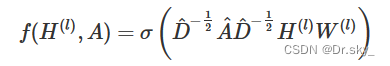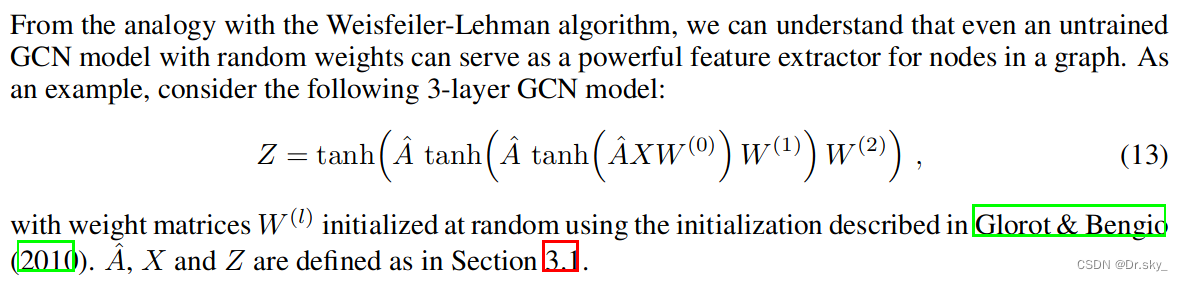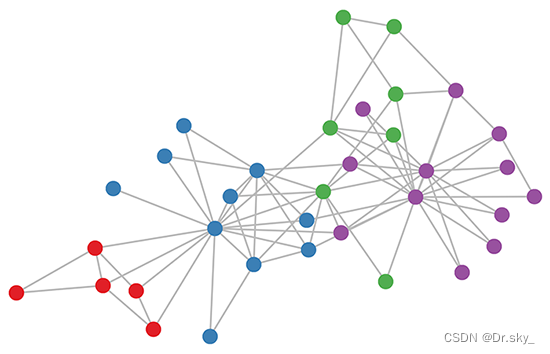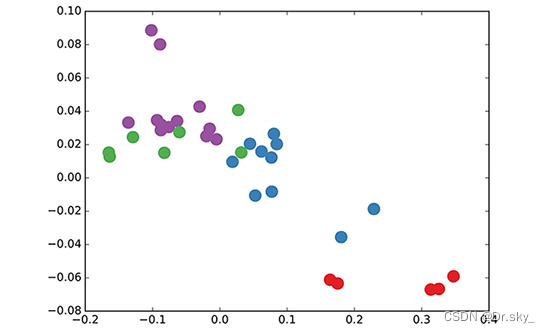1. 对于很多网络，我们可能没有节点的特征，这个时候可以使用GCN吗？答案是可以的，如论文中作者对那个俱乐部网络，采用的方法就是用单位矩阵 I 替换特征矩阵 X

2. 我没有任何的节点类别的标注，或者什么其他的标注信息，可以使用GCN吗？当然，就如前面讲的，不训练的GCN，也可以用来提取graph embedding，而且效果还不错。

3. GCN网络的层数多少比较好？论文的作者做过GCN网络深度的对比研究，在他们的实验中发现，GCN层数不宜多，2-3层的效果就很好了。

# 七、GCN的Pytorch实现

## 7.1 数据集介绍

1. 数据集结构

cora.content： 里面包含有每一篇论文各自独立的信息；

cora.cites: 里面包含有各论文之间的相互引用记录

2. 数据集内容分析

## 7.2 代码详解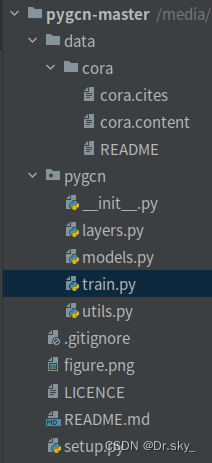1. utils.py

``import numpy as np import scipy.sparse as sp import torch  #特征独热码处理 def encode_onehot(labels):     # 将所有的标签整合成一个不重复的列表     classes = set(labels)     # set() 函数创建一个无序不重复元素集     '''enumerate()函数生成序列，带有索引i和值c。         这一句将string类型的label变为int类型的label，建立映射关系         np.identity(len(classes)) 为创建一个classes的单位矩阵         创建一个字典，索引为 label， 值为独热码向量（就是之前生成的矩阵中的某一行）'''     classes_dict = {c: np.identity(len(classes))[i, :] for i, c in                     enumerate(classes)}     # 为所有的标签生成相应的独热码     # map() 会根据提供的函数对指定序列做映射。     # 这一句将string类型的label替换为int类型的label     labels_onehot = np.array(list(map(classes_dict.get, labels)),                              dtype=np.int32)     return labels_onehot  #数据加载和处理 def load_data(path="../data/cora/", dataset="cora"):     """Load citation network dataset (cora only for now)"""     print('Loading {} dataset...'.format(dataset))     #数据包含id，features和labels     idx_features_labels = np.genfromtxt("{}{}.content".format(path, dataset),                                         dtype=np.dtype(str))     #节点的特征，维度为 2708 * 1433，类型为 np.ndarray     features = sp.csr_matrix(idx_features_labels[:, 1:-1], dtype=np.float32)     #节点的标签，总共包括7个类别，类型为 np.ndarray     labels = encode_onehot(idx_features_labels[:, -1])      # build graph     #节点的id     idx = np.array(idx_features_labels[:, 0], dtype=np.int32)     #每个id进行编号     idx_map = {j: i for i, j in enumerate(idx)}     #     edges_unordered = np.genfromtxt("{}{}.cites".format(path, dataset),                                     dtype=np.int32)     edges = np.array(list(map(idx_map.get, edges_unordered.flatten())),                      dtype=np.int32).reshape(edges_unordered.shape)   #map会根据提供的函数对指定序列做映射，flatten()就是降到一维     adj = sp.coo_matrix((np.ones(edges.shape), (edges[:, 0], edges[:, 1])),                         shape=(labels.shape, labels.shape),                         dtype=np.float32)      # build symmetric adjacency matrix     adj = adj + adj.T.multiply(adj.T > adj) - adj.multiply(adj.T > adj)      features = normalize(features)     adj = normalize(adj + sp.eye(adj.shape))      idx_train = range(140)     idx_val = range(200, 500)     idx_test = range(500, 1500)      features = torch.FloatTensor(np.array(features.todense()))     labels = torch.LongTensor(np.where(labels))     adj = sparse_mx_to_torch_sparse_tensor(adj)      idx_train = torch.LongTensor(idx_train)     idx_val = torch.LongTensor(idx_val)     idx_test = torch.LongTensor(idx_test)      return adj, features, labels, idx_train, idx_val, idx_test  #特征归一化函数 def normalize(mx):     """Row-normalize sparse matrix""" #对稀疏矩阵进行正则化处理     rowsum = np.array(mx.sum(1))      #会得到一个（2708,1）的矩阵     r_inv = np.power(rowsum, -1).flatten() #数组元素求-1次方     # 在计算倒数的时候存在一个问题，如果原来的值为0，则其倒数为无穷大，因此需要对r_inv中无穷大的值进行修正，更改为0     r_inv[np.isinf(r_inv)] = 0.     r_mat_inv = sp.diags(r_inv)     mx = r_mat_inv.dot(mx)     return mx  #精度计算函数 def accuracy(output, labels):     # 使用type_as(tesnor)将张量转换为给定类型的张量     preds = output.max(1).type_as(labels)     # 记录等于preds的label eq:equal     correct = preds.eq(labels).double()     correct = correct.sum()     return correct / len(labels)  #将scipy稀疏矩阵转换为Torch稀疏张量 def sparse_mx_to_torch_sparse_tensor(sparse_mx):     """Convert a scipy sparse matrix to a torch sparse tensor."""     """         numpy中的ndarray转化成pytorch中的tensor : torch.from_numpy()         pytorch中的tensor转化成numpy中的ndarray : numpy()         """     sparse_mx = sparse_mx.tocoo().astype(np.float32)     indices = torch.from_numpy(         np.vstack((sparse_mx.row, sparse_mx.col)).astype(np.int64))     values = torch.from_numpy(sparse_mx.data)     shape = torch.Size(sparse_mx.shape)     return torch.sparse.FloatTensor(indices, values, shape) ``

2. models.py

``import torch.nn as nn import torch.nn.functional as F from pygcn.layers import GraphConvolution   class GCN(nn.Module):     def __init__(self, nfeat, nhid, nclass, dropout):         super(GCN, self).__init__()          self.gc1 = GraphConvolution(nfeat, nhid)   #卷积层1：输入的特征为nfeat，维度是2708，输出的特征为nhid，维度是16         self.gc2 = GraphConvolution(nhid, nclass)  #卷积层2：输入的特征为nhid，维度是16，输出的特征为nclass，维度是7（即类别的结果）         self.dropout = dropout      def forward(self, x, adj):                     #forward是向前传播函数，最终得到网络向前传播的方式为：relu–>fropout–>gc2–>softmax         x = F.relu(self.gc1(x, adj))         x = F.dropout(x, self.dropout, training=self.training)         x = self.gc2(x, adj)         return F.log_softmax(x, dim=1)  ``

3. layers.py

``import math  import torch  from torch.nn.parameter import Parameter from torch.nn.modules.module import Module  #layers中主要定义了图数据实现卷积操作的层，类似于CNN中的卷积层，只是一个层而已 class GraphConvolution(Module):     """     Simple GCN layer, similar to https://arxiv.org/abs/1609.02907     """     #GraphConvolution作为一个类，首先需要定义其相关属性。主要定义了其输入特征in_feature、输出特征out_feature两个输入，     # 以及权重weight和偏移向量bias两个参数，同时调用了其参数初始化的方法     def __init__(self, in_features, out_features, bias=True):         super(GraphConvolution, self).__init__()         self.in_features = in_features         self.out_features = out_features         self.weight = Parameter(torch.FloatTensor(in_features, out_features))         if bias:             self.bias = Parameter(torch.FloatTensor(out_features))         else:             self.register_parameter('bias', None)         self.reset_parameters()      #为了让每次训练产生的初始参数尽可能的相同，从而便于实验结果的复现，可以设置固定的随机数生成种子     def reset_parameters(self):         stdv = 1. / math.sqrt(self.weight.size(1))  #标准偏差         self.weight.data.uniform_(-stdv, stdv)         if self.bias is not None:             self.bias.data.uniform_(-stdv, stdv)      #此处主要定义的是本层的前向传播，通常采用的是 A ∗ X ∗ W A * X * WA∗X∗W的计算方法。由于A是一个sparse变量，因此其与X进行卷积的结果也是稀疏矩阵     def forward(self, input, adj):         support = torch.mm(input, self.weight)         output = torch.spmm(adj, support)         if self.bias is not None:             return output + self.bias         else:             return output          #__repr__()方法是类的实例化对象用来做“自我介绍”的方法，默认情况下，它会返回当前对象的“类名+object at+内存地址”，      # 而如果对该方法进行重写，可以为其制作自定义的自我描述信息     def __repr__(self):         return self.__class__.__name__ + ' ('                 + str(self.in_features) + ' -> '                 + str(self.out_features) + ')' ``

4. train.py

``from __future__ import division from __future__ import print_function  import time import argparse import numpy as np  import torch import torch.nn.functional as F import torch.optim as optim  from pygcn.utils import load_data, accuracy from pygcn.models import GCN import matplotlib.pyplot as plt  # Training settings parser = argparse.ArgumentParser() parser.add_argument('--no-cuda', action='store_true', default=False,                     help='Disables CUDA training.') parser.add_argument('--fastmode', action='store_true', default=False,                     help='Validate during training pass.') parser.add_argument('--seed', type=int, default=42, help='Random seed.') parser.add_argument('--epochs', type=int, default=200,                     help='Number of epochs to train.') parser.add_argument('--lr', type=float, default=0.01,                     help='Initial learning rate.') parser.add_argument('--weight_decay', type=float, default=5e-4,                     help='Weight decay (L2 loss on parameters).') parser.add_argument('--hidden', type=int, default=16,                     help='Number of hidden units.') parser.add_argument('--dropout', type=float, default=0.5,                     help='Dropout rate (1 - keep probability).')  args = parser.parse_args() args.cuda = not args.no_cuda and torch.cuda.is_available()  np.random.seed(args.seed) torch.manual_seed(args.seed) if args.cuda:     torch.cuda.manual_seed(args.seed)  # Load data adj, features, labels, idx_train, idx_val, idx_test = load_data()  # Model and optimizer model = GCN(nfeat=features.shape,             nhid=args.hidden,             nclass=labels.max().item() + 1,             dropout=args.dropout) optimizer = optim.Adam(model.parameters(),                        lr=args.lr, weight_decay=args.weight_decay)  if args.cuda:     model.cuda()     features = features.cuda()     adj = adj.cuda()     labels = labels.cuda()     idx_train = idx_train.cuda()     idx_val = idx_val.cuda()     idx_test = idx_test.cuda()   def train():     loss_history = []     val_acc_history = []     t = time.time()     model.train()     if not args.fastmode:         # Evaluate validation set performance separately,         # deactivates dropout during validation run.         model.eval()         output = model(features, adj)      t_total = time.time()     for epoch in range(args.epochs):         optimizer.zero_grad()         output = model(features, adj)         loss_train = F.nll_loss(output[idx_train], labels[idx_train])         acc_train = accuracy(output[idx_train], labels[idx_train])         loss_train.backward()         optimizer.step()         loss_val = F.nll_loss(output[idx_val], labels[idx_val])         acc_val = accuracy(output[idx_val], labels[idx_val])         loss_history.append(loss_val.item())         val_acc_history.append(acc_val.item())         print('Epoch: {:04d}'.format(epoch+1),               'loss_train: {:.4f}'.format(loss_train.item()),               'acc_train: {:.4f}'.format(acc_train.item()),               'loss_val: {:.4f}'.format(loss_val.item()),               'acc_val: {:.4f}'.format(acc_val.item()),               'time: {:.4f}s'.format(time.time() - t))       print("Optimization Finished!")     print("Total time elapsed: {:.4f}s".format(time.time() - t_total))     return loss_history,val_acc_history   def test():     model.eval()     output = model(features, adj)     # test_mask_logits = output[mask]     loss_test = F.nll_loss(output[idx_test], labels[idx_test])     acc_test = accuracy(output[idx_test], labels[idx_test])     print("Test set results:",           "loss= {:.4f}".format(loss_test.item()),           "accuracy= {:.4f}".format(acc_test.item()))   #绘制loss和acc曲线 def plot_loss_with_acc(loss_history, val_acc_history):     fig = plt.figure()     ax1 = fig.add_subplot(111)     ax1.plot(range(len(loss_history)), loss_history,              c=np.array([255, 71, 90]) / 255.)     plt.ylabel('Loss')      ax2 = fig.add_subplot(111, sharex=ax1, frameon=False)     ax2.plot(range(len(val_acc_history)), val_acc_history,              c=np.array([79, 179, 255]) / 255.)     ax2.yaxis.tick_right()     ax2.yaxis.set_label_position("right")     plt.ylabel('ValAcc')      plt.xlabel('Epoch')     plt.title('Training Loss & Validation Accuracy')     plt.show()  # Train model loss, val_acc = train() plot_loss_with_acc(loss, val_acc)  #Testing test()  # 绘制测试数据的TSNE降维图 output = model(features, adj) output = output.cpu() output = output[idx_test].detach().numpy() print(output) print(output.shape) from sklearn.manifold import TSNE tsne = TSNE() out = tsne.fit_transform(output) fig = plt.figure() labels_test = labels[idx_test].detach().cpu().numpy() for i in range(7):     indices = labels_test == i     # print(indices)     x, y = out[indices].T     plt.scatter(x, y, label=str(i)) plt.legend() plt.show() ``

5. 代码运行结果

（1）loss和acc训练曲线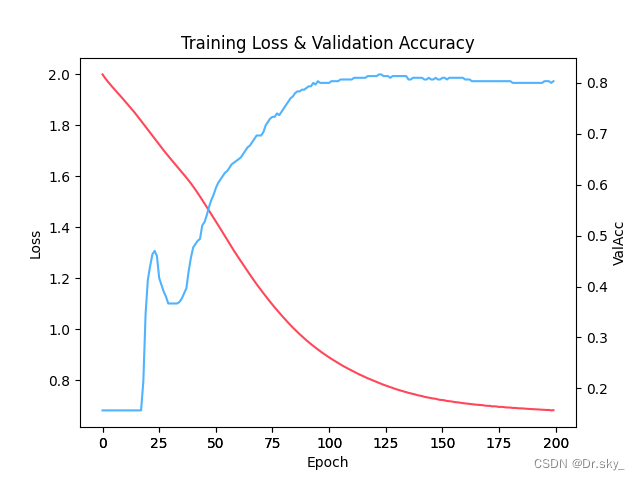（2）测试数据的TSNE降维图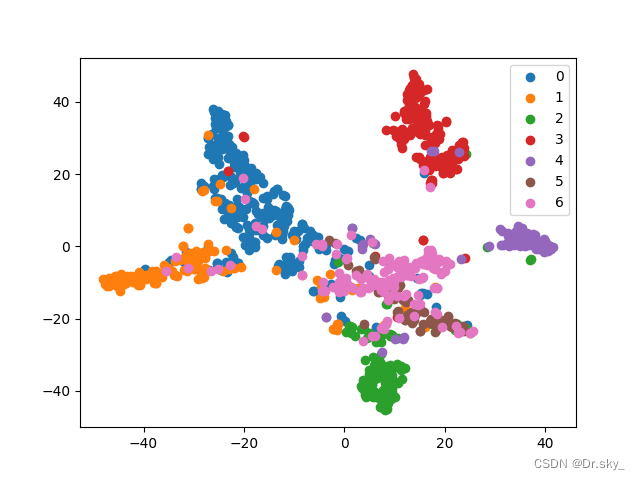3.

4.

5.